## Molecule of the Month - October 1997# N2S2 - Disulfur Dinitride## J. Gerratt and S.J. McNicholas

### School of Chemistry, University of Bristol

This version is the plain HTML version with no plug-ins or embedded molecular structures.
A version using the Chemscape Chime plug-in can be found here, but you will need to install the plug-in beforehand to view this page properly.

The nature of the bonding in N2S2 (and other N-S compounds) is far from obvious. A favourite first year University examination question is 'N2S2 is said to be aromatic. Discuss'. The answer is meant to be that the molecule is planar and possesses six electrons in orbitals of p symmetry, which implies some similarity to the benzene molecule. In actual fact, nothing could be further from the truth.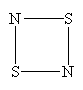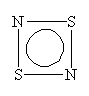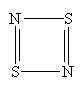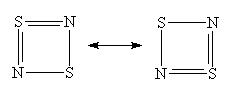So what is the correct bonding and structure of N2S2? According to Greenwood and Earnshaw , the geometry of the N2S2 molecule is, indeed, almost exactly a square, in spite of the disparity in the sizes of the S and N atoms: The S-N bond lengths, determined from X-ray diffraction studies, are 165.1 and 165.7 pm, the S-N-S bond angle is 90.4°, while the N-S-N angle is 89.9°. At room temperature, N2S2 readily polymerizes to form (SN)x, which is metallic. At very low temperatures (0.33K), the polymer becomes superconducting. There is consequently much about the electronic structure of this system to warrant a close study of the N2S2 monomer itself.

We can calculate the structure of molecules such as N2S2 using the spin-coupled valence bond method. The spin-coupled wave function incorporates much chemically significant electron correlation in a compact and highly visual form. This approach to the determination of molecular electronic structure is described in detail in several places (see e.g.). The six p orbitals, which are each singly-occupied are shown in Figure 1 below (see ):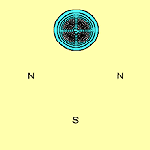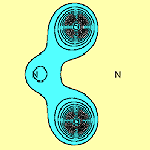f1 f2 f3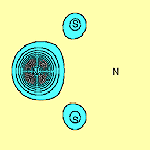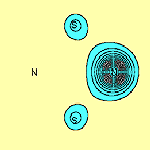f4 f5 f6

The contours are plotted in a plane parallel to that of the molecule and 1 bohr above it. From this, we see immediately that on each of the two S atoms there is indeed only a single p orbital: orbitals f1 and f2 respectively. It can be seen that they are well localized. Each N atom also has a single highly localized p orbital centred upon it (orbitals f4 and f5 respectively). In addition to this, there are two three-centre p orbitals, symmetrically related to each other, centred about each N atom and stretching over the two S-N-S subsystems (orbitals f3 and f6). The sulfur atoms bear a significant positive charge, +0.52e in the DZP basis, and the nitrogen atoms a complementary negative charge.

A transposition of orbitals f1 and f2, the p orbitals on the S atoms, is equivalent to a symmetry operation of the molecule: i.e. the transposition is equivalent to a reflection in a plane perpendicular to that of the molecule and passing through both N atoms. Since the total electronic wave function for the ground state, Y00, belongs to the totally symmetric representation Ag of D2h, it must remain invariant under this operation. Consequently Y00 must be symmetric towards interchange of the spatial coordinates of the two electrons described by f1 and f2. This means that in a state of symmetry Ag in D2h, the spins of the electrons in f1 and f2 must be coupled exactly to a singlet. The 'perfectly paired' spin function, according to which the spins of orbital pairs (f1,f2), (f3,f4) and (f5,f6), are to an excellent approximation simply paired up in singlets, predominates heavily.

From an initial glance at these results, one might conclude that there is a direct S-S p bond. However further examination of orbitals f1 and f2, (i.e. in a plane perpendicular to the molecular plane and passing through both S atoms), shows that this is not so, as can be seen in Figure 2.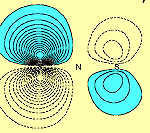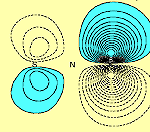f1 f2

Orbitals f1 and f2 each possess a nodal surface, roughly half-way between the two S atoms, the lobes of f1 and f2 are bent slightly away from the other S-atom partner and the total electron density actually decreases within the N2S2 ring. These nodal surfaces originate from the radial nodal surfaces present in the 3p orbitals of sulphur.

Normally when two orbitals on different atoms overlap and the spins of the electrons occupying each of them are paired up, we consider this to be a single bond. Otherwise if the two orbitals happen to be orthogonal, either exactly by symmetry, or effectively so due to the distance between them, the lowest state is usually obtained when the electron spins are coupled to a triplet. The molecule is then referred to as a diradical. In N2S2, a different situation arises: Orbitals f1 and f2 overlap and the corresponding spins are coupled to a singlet. Yet no true bond is formed. We therefore consider that N2S2 in its ground electronic state is best described as a singlet diradical.

A moment's thought will convince one that this bonding pattern is in accord with all the valencies within this single 'spin-coupled structure', though in a highly unexpected manner: N- ions have a valency of two and accordingly form two single bonds of s symmetry with a bond angle between them of 90°, one with each of the neighbouring S atoms. The S+ ions have a valency of three and accordingly they each form two bonds of s symmetry with adjacent N- ions. We thus have a s single bond framework for N2S2. The remaining two electrons each occupy a single p orbital on each S atom and are singlet coupled to each other. Because the orbitals involved are purely p in character, the question of bond angles does not arise. N2S2 can therefore be represented reasonably well by the bonding scheme shown in Figure 3 in which the dotted line joining the two S atoms indicates the singlet diradical character of the link.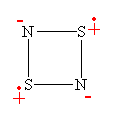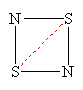## (SN)x - a conducting polymer

N2S2 can explode, and is also thermally pyrolised to give the polymeric (SN)x, which is electrically conducting. Can we explain this metallic behaviour on the basis of its bonding? The geometric structure of the (SN)x chain, which is not completely flat, is shown in Figure 4.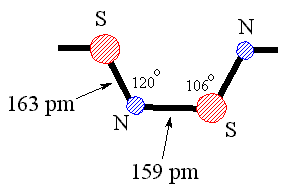Figure 4. A section of the conducting polymer (SN)x. Click on the images to view the pdb file.

We have carried out calculations on N2S2 in which two adjacent angles, SNS and NSN are opened out to 120° and 106°, thus effectively breaking an N-S bond. The resulting p orbitals are shown in Figure 5. It can be seen that a singly-occupied p orbital on each S atom, f1 and f2, remains. In addition, the two orbitals forming the lone pair centred on the S-N-S group, f3 and f4, in which f4 is tightly bound around the N atom, the other, f3, is three-centred, also survive. However, the lone pair associated with what is now the terminal N atom, is different: The orbital, f6 which in N2S2 is three-centred, is now only two-centred.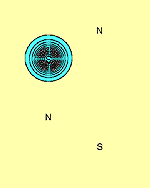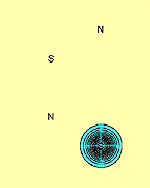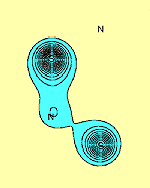f1 f2 f3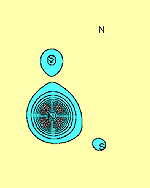f4 f5 f6

We thus see that the (SN)x chain appears to consist, besides the electrons forming the s bonds which hold it together, of a singly-occupied p orbital on each S atom, interspersed with a p lone pair centred around each N atom. The polymer may therefore to a good approximation be regarded as a one-dimensional chain of S atoms, with a single electron on each site. This is equivalent to a half-filled band and consequently one would predict the polymer to be metallic, in agreement with observation.

### References

1. N.N. Greenwood and A. Earnshaw, Chemistry of the Elements, Pergamon Press, Oxford 1984.
2. J. Gerratt, S.J. McNicholas, M.Sironi, D.L. Cooper and P.B. Karadakov, 'The extraordinary electronic structure and bonding of N2S2', J. Am. Chem. Soc. 118 (1996) 6472-6476.
3. J. Gerratt, D.L. Cooper, P.B. Karadakov and M. Raimondi 'Modern Valence Bond Theory' Chemical Society Reviews, 26, (1997) 87-100.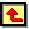Back to Molecule of the Month page.        [DOI:10.6084/m9.figshare.5469559]